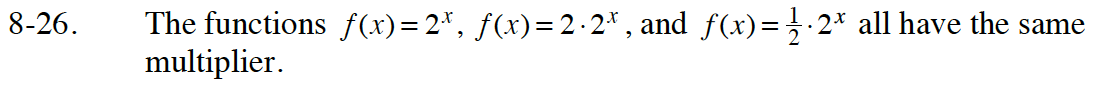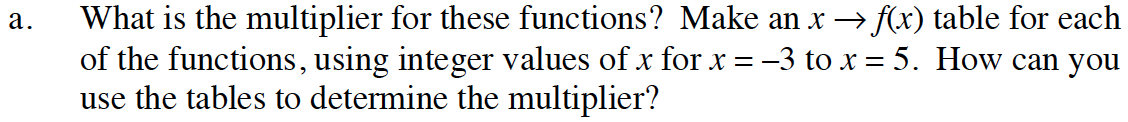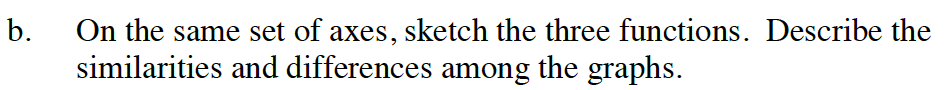### Home > INT1 > Chapter Ch8 > Lesson 8.1.2 > Problem8-26

8-26.
1. The functions f(x) = 2x, f(x) = 2 · 2x, and f(x) =· 2x all have the same multiplier. 8-26 HW eTool (Desmos). Homework Help ✎

1. What is the multiplier for these functions? Make an xf(x) table for each of the functions, using integer values of x for x = −3 to x = 5. How can you use the tables to determine the multiplier?

2. On the same set of axes, sketch the three functions. Describe the similarities and differences among the graphs.For example, compute f(1) and f(2). What number do you need to multiply f(1) by to get f(2)?Compare the general shapes, intercepts, asymptotes, and growth rates.

Use the eTool below to help solve for each part.
Click the link to the right to view full version: Int1 8-26 HW eTool.